Browse by Topic

# Limits without Limits in Version 11.2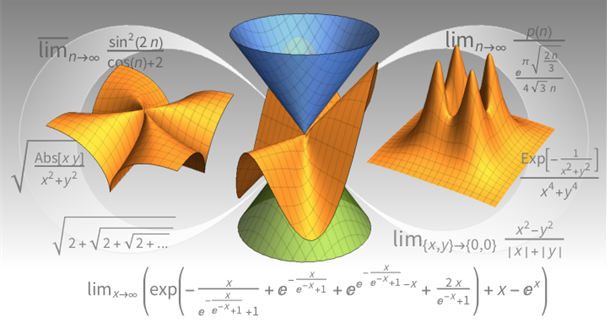Here are 10 terms in a sequence: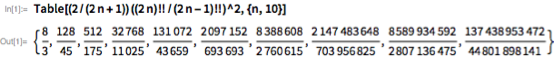And here’s what their numerical values are: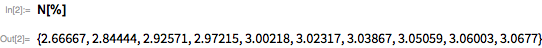But what is the limit of the sequence? What would one get if one continued the sequence forever?

In Mathematica and the Wolfram Language, there’s a function to compute that: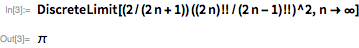Limits are a central concept in many areas, including number theory, geometry and computational complexity. They’re also at the heart of calculus, not least since they’re used to define the very notions of derivatives and integrals.

Mathematica and the Wolfram Language have always had capabilities for computing limits; in Version 11.2, they’ve been dramatically expanded. We’ve leveraged many areas of the Wolfram Language to achieve this, and we’ve invented some completely new algorithms too. And to make sure we’ve covered what people want, we’ve sampled over a million limits from Wolfram|Alpha.

Let’s talk about a limit that Hardy and Ramanujan worked out in 1918. But let’s build up to that. First, consider the sequence a(n) that is defined as follows: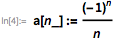Here is a table of the first ten values for the sequence.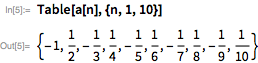The following plot indicates that the sequence converges to 0 as n approaches Infinity.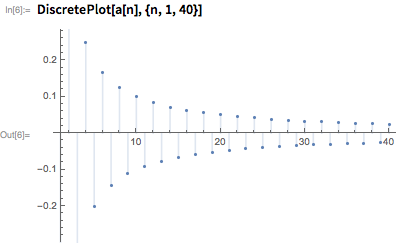The DiscreteLimit function, which was introduced in Version 11.2, confirms that the limit of this sequence is indeed 0.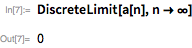Many sequences that arise in practice (for example, in signal communication) are periodic in the sense that their values repeat themselves at regular intervals. The length of any such interval is called the period of the sequence. As an example, consider the following sequence that is defined using Mod.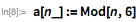A plot of the sequence shows that the sequence is periodic with period 6.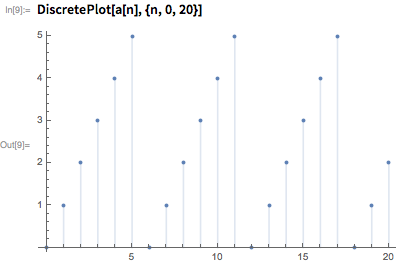In contrast to our first example, this sequence does not converge, since it oscillates between 0 and 5. Hence, DiscreteLimit returns Indeterminate in this case.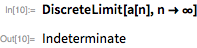The new Version 11.2 functions DiscreteMinLimit and DiscreteMaxLimit can be used to compute the lower and upper limits of oscillation, respectively, in such cases. Thus, we have: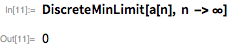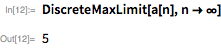DiscreteMinLimit and DiscreteMaxLimit are often referred to as “lim inf” and “lim sup,” respectively, in the mathematical literature. The traditional underbar and overbar notations for these limits are available, as shown here.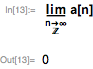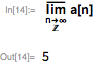Our next example is an oscillatory sequence that is built from the trigonometric functions Sin and Cos, and is defined as follows.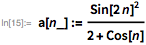Although Sin and Cos are periodic when viewed as functions over the real numbers, this integer sequence behaves in a bizarre manner and is very far from being a periodic sequence, as confirmed by the following plot.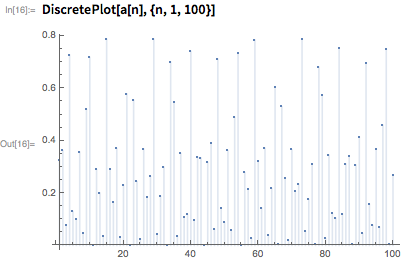Hence, the limit of this sequence does not exist.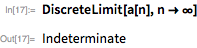However, it turns out that for such “densely aperiodic sequences,” the extreme values can be computed by regarding them as real functions. DiscreteMinLimit uses this method to return the answer 0 for the example, as expected.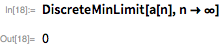Using the same method, DiscreteMaxLimit returns a rather messy-looking result in terms of Root objects for this example.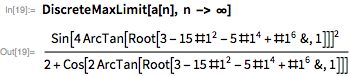The numerical value of this result is close to 0.8, as one might have guessed from the graph.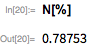Discrete limits also occur in a natural way when we try to compute the value of infinitely nested radicals. For example, consider the problem of evaluating the following nested radical.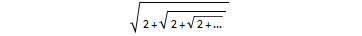The successive terms in the expansion of the radical can be generated by using RSolveValue, since the sequence satisfies a nonlinear recurrence. For example, the third term in the expansion is obtained as follows.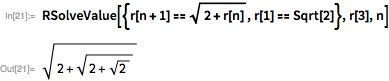The value of the infinitely nested radical appears to be 2, as seen from the following plot that is generated using RecurrenceTable.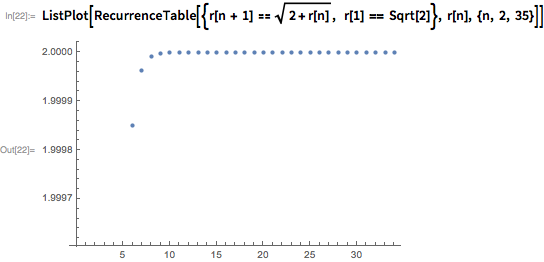Using Version 11.2, we can confirm that the limiting value is indeed 2 by requesting the value r(∞) in RSolveValue, as shown here.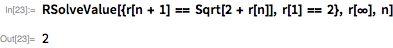The study of limits belongs to the branch of mathematics called asymptotic analysis. Asymptotic analysis provides methods for obtaining approximate solutions of problems near a specific value such as 0 or Infinity. It turns out that, in practice, the efficiency of asymptotic approximations often increases precisely in the regime where the corresponding exact computation becomes difficult! A striking example of this phenomenon is seen in the study of integer partitions, which are known to grow extremely fast as the size of the number increases. For example, the number 6 can be partitioned in 11 distinct ways using IntegerPartitions, as shown here.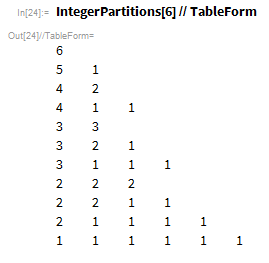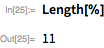The number of distinct partitions can be found directly using PartitionsP as follows.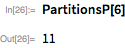As noted earlier, the number of partitions grows rapidly with the size of the integer. For example, there are nearly 4 trillion partitions of the number 200.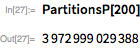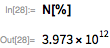In 1918, Hardy and Ramanujan provided an asymptotic approximation for this number, which is given by the following formula.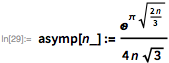The answer given by this estimate for the number 200 is remarkably close to 4 trillion.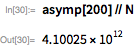With a much larger integer, we get an even better approximation for the number of partitions almost instantaneously, as seen in the following example.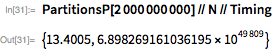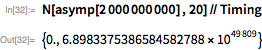Finally, we can confirm that the asymptotic estimate approaches the number of partitions as n tends to Infinity using DiscreteLimit, which is aware of the Hardy–Ramanujan formula discussed above.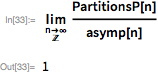Formally, we say that exact and approximate formulas for the number of partitions are asymptotically equivalent as n approaches Infinity.

Asymptotic notions also play an important rule in the study of function limits. For instance, the small-angle approximation in trigonometry asserts that “sin(x) is nearly equal to x for small values of x.” This may be rephrased as “sin(x) is asymptotically equivalent to x as x approaches 0.” A formal statement of this result can be given using Limit, which computes function limits, as follows.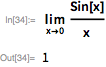This plot provides visual confirmation that the limit is indeed 1.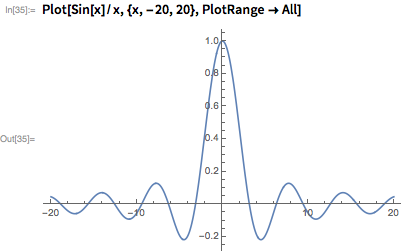The above limit can also be calculated using L’Hôspital’s rule by computing the derivatives, cos(x) and 1, of the numerator and denominator respectively, as shown here.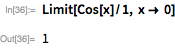L’Hôspital’s rule gives a powerful method for evaluating many limits that occur in practice. However, it may require a large number of steps before arriving at the answer. For example, consider the following limit.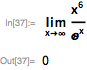That limit requires six repeated applications of L’Hôspital’s rule to arrive at the answer 0, since all the intermediate computations give indeterminate results.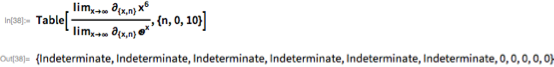Thus, we see that L’Hôspital’s rule has limited utility as a practical algorithm for finding function limits, since it is impossible to decide when the algorithm should stop! Hence, the built-in Limit function uses a combination of series expansions and modern algorithms that works well on inputs involving exponentials and logarithms, the so-called “exp-log” class. In fact, Limit has received a substantial update in Version 11.2 and now handles a wide variety of difficult examples, such as the following, in a rather comprehensive manner (the last two examples work only in the latest release).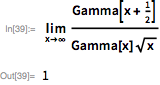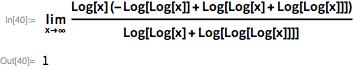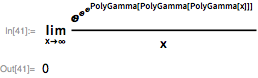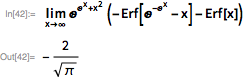As in the cases of sequences, the limits of periodic and oscillatory functions will often not exist. One can then use MaxLimit and MinLimit, which, like their discrete counterparts, give tight bounds for the oscillation of the function near a given value, as in this classic example.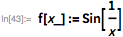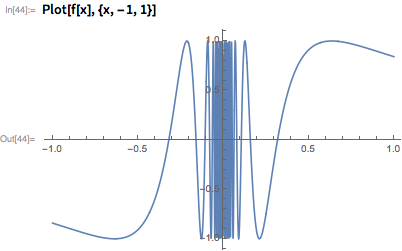The graph indicates the function oscillates rapidly between –1 and 1 near 0. These bounds are confirmed by MaxLimit and MinLimit, while Limit itself returns Indeterminate.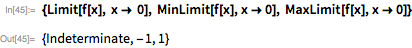In the previous example, the limit fails to exist because the function oscillates wildly around the origin. Discontinuous functions provide other types of examples where the limit at a point may fail to exist. We will now consider an example of such a function with a jump discontinuity at the origin and other values. The function is defined in terms of SquareWave and FresnelS, as follows.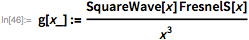This plot shows the jump discontinuities, which are caused by the presence of SquareWave in the definition of the function.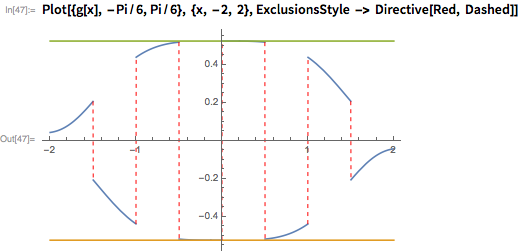We see that the limiting values of the function at 0, for instance, depend on the direction from which we approach the origin. The limiting value from the right (“from above”) can be calculated using the Direction option.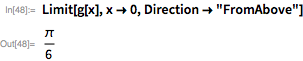Similarly, the limit from the left can be calculated as follows.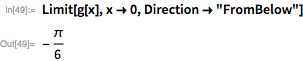The limit, if it exists, is the “two-sided” limit for the function that, in this case, does not exist.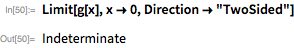By default, Limit computes two-sided limits in Version 11.2. This is a change from earlier versions, where it computed the limit from above by default. Hence, we get an Indeterminate result from Limit, with no setting for the Direction option.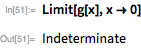Directional limits acquire even more significance in the multivariate case, since there are many possible directions for approaching a given point in higher dimensions. For example, consider the bivariate function f(x,y) that is defined as follows.The limiting value of this function at the origin is 0 if we approach it along the x axis, which is given by y=0, since the function has the constant value 0 along this line.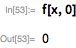Similarly, the limiting value of the function at the origin is 0 if we approach it along the y axis, which is given by x=0.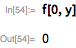However, the limit is 1/2 if we approach the origin along the line y=x, as seen here.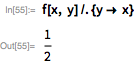More generally, the limiting value changes as we approach the origin along different lines y=m x.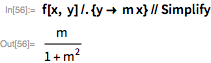The directional dependence of the limiting value implies that the true multivariate limit does not exist. In Version 11.2, Limit handles multivariate examples with ease, and quickly returns the expected answer Indeterminate for the limit of this function at the origin.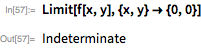A plot of the surface z=f(x,y) confirms the behavior of the function near the origin.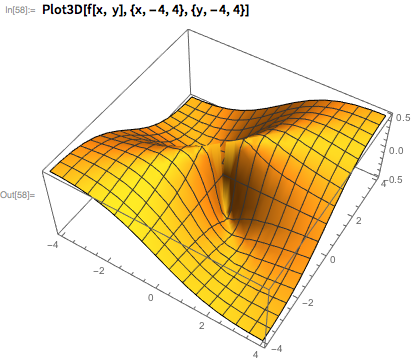This example indicates that, in general, multivariate limits do not exist. In other cases, such as the following, the limit exists but the computation is subtle.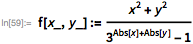This plot indicates that the limit of this function at {0,0} exists and is 0, since the function values appear to approach 0 from all directions.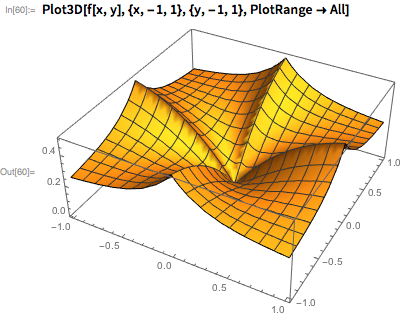The answer can be confirmed by applying Limit to the function directly.A rich source of multivariate limit examples is provided by the steady stream of inputs that is received by Wolfram|Alpha each day. We acquired around 100,000 anonymized queries to Wolfram|Alpha from earlier years, which were then evaluated using Version 11.2 . Here is a fairly complicated example from this vast collection that Limit handles with ease in the latest version.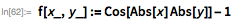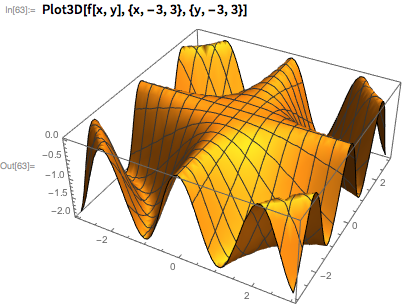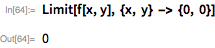It is a sheer joy to browse through the examples from Wolfram|Alpha, so we decided to share 1,000 nontrivial examples from the collection with you. Sample images of the examples are shown below. The five notebooks with the examples can be downloaded here.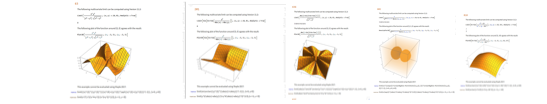Version 11.2 evaluates 90% of the entire collection in the benchmark, which is remarkable since the functionality for multivariate limits is new in this release.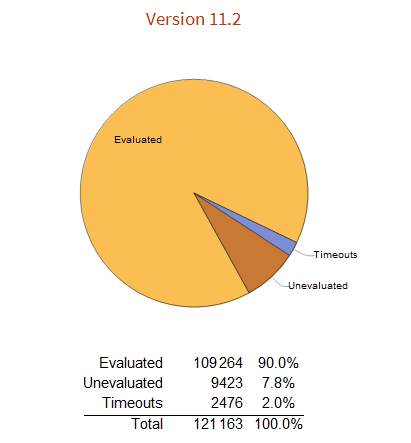Version 11.2 also evaluates a higher fraction (96%) of an even larger collection of 1,000,000 univariate limits from Wolfram|Alpha when compared with Version 11.1 (94%). The small percentage difference between the two versions can be explained by noting that most Wolfram|Alpha queries for univariate limits relate to a first or second course in college calculus and are easily computed by Limit in either version.

Limit has been one of the most dependable functions in the Wolfram Language ever since it was first introduced in Version 1 (1988). The improvements for this function, along with DiscreteLimit and other new functions in Version 11.2, have facilitated our journey through the world of limits. I hope that you have enjoyed this brief tour, and welcome any comments or suggestions about the new features.